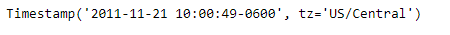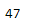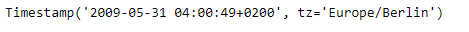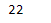Related Articles

# Python | Pandas Timestamp.week

• Last Updated : 08 Jan, 2019

Python is a great language for doing data analysis, primarily because of the fantastic ecosystem of data-centric python packages. Pandas is one of those packages and makes importing and analyzing data much easier.

Pandas` Timestamp.week` attribute return an integer value which is the ordinal value of the week in which the date of the given Timestamp object lies.

Syntax : Timestamp.week

Parameters : None

Return : week

Example #1: Use `Timestamp.week` attribute to find the ordinal value of the week in which the date of the given Timestamp object lies.

 `# importing pandas as pd``import` `pandas as pd`` ` `# Create the Timestamp object``ts ``=` `pd.Timestamp(year ``=` `2011``,  month ``=` `11``, day ``=` `21``,``           ``hour ``=` `10``, second ``=` `49``, tz ``=` `'US/Central'``)`` ` `# Print the Timestamp object``print``(ts)`

Output :Now we will use the `Timestamp.week` attribute to find ordinal value of the week

 `# return the week number``ts.week`

Output :As we can see in the output, the `Timestamp.week` attribute has returned 47 indicating that the date in the give Timestamp object falls in the 47th week of the year.

Example #2: Use `Timestamp.week` attribute to find the ordinal value of the week in which the date of the given Timestamp object lies.

 `# importing pandas as pd``import` `pandas as pd`` ` `# Create the Timestamp object``ts ``=` `pd.Timestamp(year ``=` `2009``,  month ``=` `5``, day ``=` `31``,``        ``hour ``=` `4``, second ``=` `49``, tz ``=` `'Europe/Berlin'``)`` ` `# Print the Timestamp object``print``(ts)`

Output :Now we will use the `Timestamp.week` attribute to find ordinal value of the week

 `# return the week number``ts.week`

Output :As we can see in the output, the `Timestamp.week` attribute has returned 22 indicating that the date in the give Timestamp object falls in the 22nd week of the year.

Attention geek! Strengthen your foundations with the Python Programming Foundation Course and learn the basics.

To begin with, your interview preparations Enhance your Data Structures concepts with the Python DS Course. And to begin with your Machine Learning Journey, join the Machine Learning – Basic Level Course

My Personal Notes arrow_drop_up